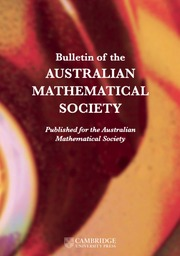Skip to main content Accessibility help
Home
Hostname: page-component-564cf476b6-scc96 Total loading time: 0.217 Render date: 2021-06-23T06:46:47.433Z Has data issue: true Feature Flags: { "shouldUseShareProductTool": true, "shouldUseHypothesis": true, "isUnsiloEnabled": true, "metricsAbstractViews": false, "figures": true, "newCiteModal": false, "newCitedByModal": true, "newEcommerce": true }Bulletin of the Australian Mathematical Society

## Abstract

We study the fractional parabolic Lichnerowicz equation

\begin{align*} v_t+(-\Delta)^s v=v^{-p-2}-v^p \quad\mbox{in } \mathbb R^N\times\mathbb R \end{align*}where$p>0$ and$0<s<1$. We establish a Liouville-type theorem for positive solutions in the case$p>1$ and give a uniform lower bound of positive solutions when$0<p\leq 1$. In particular, when v is independent of the time variable, we obtain a similar result for the fractional elliptic Lichnerowicz equation

\begin{align*} (-\Delta)^s u=u^{-p-2}-u^p \quad\mbox{in }\mathbb R^N \end{align*}with$p>0$ and$0<s<1$. This extends the result of Brézis [‘Comments on two notes by L. Ma and X. Xu’, C. R. Math. Acad. Sci. Paris 349(5–6) (2011), 269–271] to the fractional Laplacian.

## MSC classification

Type
Research Article
Copyright
©2021 Australian Mathematical Publishing Association Inc.

## Access options

Get access to the full version of this content by using one of the access options below.

## Footnotes

The research of A. T. Duong is funded by the Vietnam Ministry of Education and Training under grant number B2021-SPH-15.

## References

Brezis, H., ‘Comments on two notes by L. Ma and X. Xu’, C. R. Math. Acad. Sci. Paris 349(5–6) (2011), 269271.CrossRefGoogle Scholar
Chen, H. and Véron, L., ‘Initial trace of positive solutions to fractional diffusion equations with absorption’, J. Funct. Anal. 276(4) (2019), 11451200.CrossRefGoogle Scholar
Choquet-Bruhat, Y., Isenberg, J. and Pollack, D., ‘The Einstein-scalar field constraints on asymptotically Euclidean manifolds’, Chinese Ann. Math. Ser. B 27(1) (2006), 3152.CrossRefGoogle Scholar
Choquet-Bruhat, Y., Isenberg, J. and Pollack, D., ‘The constraint equations for the Einstein-scalar field system on compact manifolds’, Classical Quantum Gravity 24(4) (2007), 809828.CrossRefGoogle Scholar
Dung, N. T., Khanh, N. N. and Ngô, Q. A., ‘Gradient estimates for some$f$-heat equations driven by Lichnerowicz’s equation on complete smooth metric measure spaces’, Manuscripta Math. 155(3–4) (2018), 471501.CrossRefGoogle Scholar
Duong, A. T. and Nguyen, V. H., ‘A Liouville type theorem for fractional elliptic equation with exponential nonlinearity’, Preprint, arXiv:1911.05966, 2019.Google Scholar
Ferrari, F. and Verbitsky, I. E., ‘Radial fractional Laplace operators and Hessian inequalities’, J. Differential Equations 253(1) (2012), 244272.CrossRefGoogle Scholar
Frank, R. L., Lenzmann, E. and Silvestre, L., ‘Uniqueness of radial solutions for the fractional Laplacian’, Comm. Pure Appl. Math. 69(9) (2016), 16711726.CrossRefGoogle Scholar
Hebey, E., Pacard, F. and Pollack, D., ‘A variational analysis of Einstein-scalar field Lichnerowicz equations on compact Riemannian manifolds’, Comm. Math. Phys. 278(1) (2008), 117132.CrossRefGoogle Scholar
Ma, L., ‘Liouville type theorem and uniform bound for the Lichnerowicz equation and the Ginzburg–Landau equation’, C. R. Math. Acad. Sci. Paris 348(17–18) (2010), 993996.CrossRefGoogle Scholar
Ma, L., ‘Boundedness of solutions to Ginzburg–Landau fractional Laplacian equation’, Internat. J. Math. 27(5) (2016), 1650048, 6 pages.CrossRefGoogle Scholar
Ma, L. and Xu, X., ‘Uniform bound and a non-existence result for Lichnerowicz equation in the whole$n$-space’, C. R. Math. Acad. Sci. Paris 347(13–14) (2009), 805808.CrossRefGoogle Scholar
Molica Bisci, G., V. Radulescu, D. and Servadei, R., Variational Methods for Nonlocal Fractional Problems, Encyclopedia of Mathematics and its Applications, 162 (Cambridge University Press, Cambridge, 2016).CrossRefGoogle Scholar
Zhao, L., ‘Harnack inequality for parabolic Lichnerowicz equations on complete noncompact Riemannian manifolds’, Bound. Value Probl. 2013 (2013), 190.CrossRefGoogle Scholar
Zhao, L., ‘Gradient estimates for a simple parabolic Lichnerowicz equation’, Osaka J. Math. 51(1) (2014), 245256.Google Scholar

# Send article to Kindle

To send this article to your Kindle, first ensure no-reply@cambridge.org is added to your Approved Personal Document E-mail List under your Personal Document Settings on the Manage Your Content and Devices page of your Amazon account. Then enter the ‘name’ part of your Kindle email address below. Find out more about sending to your Kindle. Find out more about sending to your Kindle.

Note you can select to send to either the @free.kindle.com or @kindle.com variations. ‘@free.kindle.com’ emails are free but can only be sent to your device when it is connected to wi-fi. ‘@kindle.com’ emails can be delivered even when you are not connected to wi-fi, but note that service fees apply.

Find out more about the Kindle Personal Document Service.

UNIFORM LOWER BOUND AND LIOUVILLE TYPE THEOREM FOR FRACTIONAL LICHNEROWICZ EQUATIONS
Available formats
×

# Send article to Dropbox

To send this article to your Dropbox account, please select one or more formats and confirm that you agree to abide by our usage policies. If this is the first time you use this feature, you will be asked to authorise Cambridge Core to connect with your <service> account. Find out more about sending content to Dropbox.

UNIFORM LOWER BOUND AND LIOUVILLE TYPE THEOREM FOR FRACTIONAL LICHNEROWICZ EQUATIONS
Available formats
×

# Send article to Google Drive

To send this article to your Google Drive account, please select one or more formats and confirm that you agree to abide by our usage policies. If this is the first time you use this feature, you will be asked to authorise Cambridge Core to connect with your <service> account. Find out more about sending content to Google Drive.

UNIFORM LOWER BOUND AND LIOUVILLE TYPE THEOREM FOR FRACTIONAL LICHNEROWICZ EQUATIONS
Available formats
×
×

#### Reply to:Submit a response

Please enter your response.

#### Your details

Please enter a valid email address.

#### Conflicting interests

Do you have any conflicting interests? *